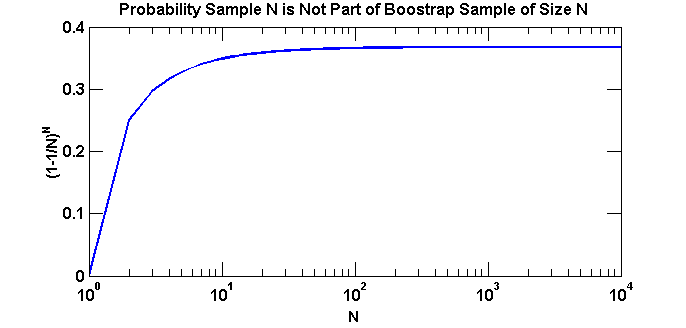-->

## Tuesday, October 15, 2013

### What's the Chance an Example will be Chosen from a Bootstrap Sample?

I've been studying Random Forest classifiers for fun and profit. They have many advantages, work fairly well and are easy to tune. One of the features of a RF classifier is that it is an ensemble of tree-based classifiers, where each tree is learned using a bootstrap sample (with replacement) of the data.
If we pretend our data is like a big pool of numbered balls, we draw one ball from the pool and copy the number to another ball that we set aside. Now, toss the ball back, mix, pick another ball and repeat. In this scheme you have a chance of drawing the same ball twice (or more). This probability is $\frac{1}{N}^D$, where D is the number of draws, if the balls are well mixed.
As I was reading Leo Brieman's description of the algorithm (which is very readable), I noticed the remark, "About one-third of the cases are left out of the bootstrap sample and not used in the construction of the kth tree". Is that true?
If the probability of chosing one sample at random is $1/N$, then the probability that a sample is not chosen is $1-\frac{1}{N}$. For independent draws, the chance that a sample is not chosen twice is
\begin{aligned} \left(1- \frac{1}{N}\right)\left(1- \frac{1}{N}\right) = \left(1- \frac{1}{N}\right)^2. \end{aligned}
For $D$ independent draws the probably of not chosing that sample is
\begin{aligned} \left(1- \frac{1}{N}\right)^D \end{aligned}
Hmmmm ... that looks interesting. Suppose we let $D$ go to $N$, meaning if we have 10 total samples we draw 10 "boostrap samples". What's the probability that we'll miss a sample? Wolfram|Alpha can make this a bit easier. Turns that as we go to a very large dataset, $N\to\infty$ this converges to something specific:
\begin{aligned} \lim_{N\to\infty }(1-\frac{1}{N})^N=\frac{1}{e}\approx 0.36787... \end{aligned}
Pretty interesting. This means that the probability a sample will be chosen is $\approx 0.64$, which justifies Brieman's statement that, "About one-third of the cases are left out". Pictorally this looks like: